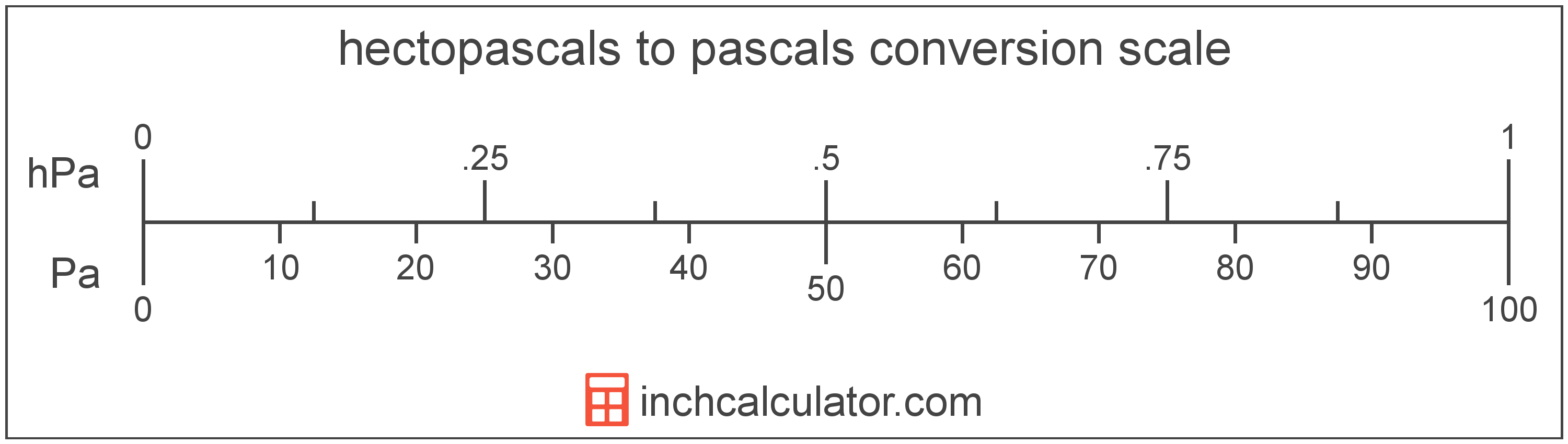# Pascals to Hectopascals Converter

Enter the pressure in pascals below to get the value converted to hectopascals.

Results in Hectopascals:1 Pa = 0.01 hPa

Do you want to convert hectopascals to pascals?

## How to Convert Pascals to Hectopascals

To convert a measurement in pascals to a measurement in hectopascals, divide the pressure by the following conversion ratio: 100 pascals/hectopascal.

Since one hectopascal is equal to 100 pascals, you can use this simple formula to convert:

hectopascals = pascals ÷ 100

The pressure in hectopascals is equal to the pressure in pascals divided by 100.

For example, here's how to convert 500 pascals to hectopascals using the formula above.
hectopascals = (500 Pa ÷ 100) = 5 hPa## What is a Pascal?

The pascal is defined as the pressure of one newton per square meter.

The pascal is the SI derived unit for pressure in the metric system. Pascals can be abbreviated as Pa; for example, 1 pascal can be written as 1 Pa.

Pascals can be expressed using the formula:
1 Pa = 1 N / m2

Pressure in pascals is equal to the force in newtons divided by the area in square meters.

## What is a Hectopascal?

The hectopascal is equal to 100 pascals, which are defined as the pressure of one newton per square meter. The hectopascal is the internationally adopted unit used to measure atmospheric pressure.

The hectopascal is a multiple of the pascal, which is the SI derived unit for pressure. In the metric system, "hecto" is the prefix for 102. Hectopascals can be abbreviated as hPa; for example, 1 hectopascal can be written as 1 hPa.

## Pascal to Hectopascal Conversion Table

Table showing various pascal measurements converted to hectopascals.
Pascals Hectopascals
1 Pa 0.01 hPa
2 Pa 0.02 hPa
3 Pa 0.03 hPa
4 Pa 0.04 hPa
5 Pa 0.05 hPa
6 Pa 0.06 hPa
7 Pa 0.07 hPa
8 Pa 0.08 hPa
9 Pa 0.09 hPa
10 Pa 0.1 hPa
20 Pa 0.2 hPa
30 Pa 0.3 hPa
40 Pa 0.4 hPa
50 Pa 0.5 hPa
60 Pa 0.6 hPa
70 Pa 0.7 hPa
80 Pa 0.8 hPa
90 Pa 0.9 hPa
100 Pa 1 hPa